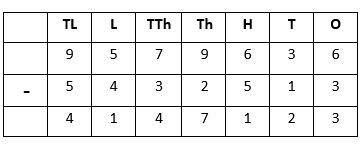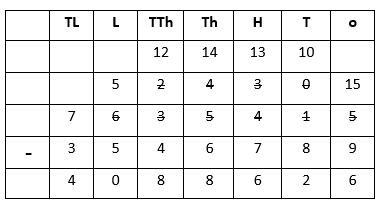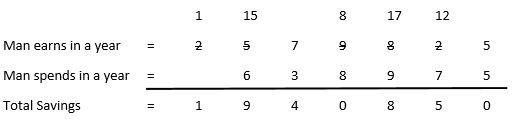## LetsPlayMaths.Com

WELCOME TO THE WORLD OF MATHEMATICS

# Class 4 Subtraction

Subtraction of 6-Digit and 7-Digit Numbers Without Borrowing

Subtraction of 6-Digit and 7-Digit Numbers With Borrowing

Story Time Subtraction

Subtraction Test

Subtraction Worksheet

Answer Sheet

## Subtraction of 6-Digit and 7-Digit Numbers Without Borrowing

In class III we have learned how to subtract 4-digit and 5-digit numbers. Now let us subtract 6-digit and 7-digit numbers using the same method.

6-digit and 7-digit numbers are subtracted in the same way as smaller numbers. First, we subtract the digits in the ones place, then we subtract the digits in the tens place, followed by those in hundreds, thousands, ten thousands and lakhs places.

Example 1. Subtract 246587 from 879898.

Solution.
O - Ones
T - Tens
H - Hundred
Th - Thousands
T-Th - Ten Thousand
L- Lakh
TL - Ten LakhSo, the result is 633311.

Example 2. Subtract 5432513 from 9579636.

Solution.So, the result is 4147123.

## Subtraction of 6-Digit and 7-Digit Numbers With Borrowing

In this kind of subtraction, we use the same method as above. But, we borrow numbers from the next higher place value wherever required.

Example 1. Subtract 275428 from 542376.

Solution.So, the result is 266948.

Example 2. Subtract 3546789 from 7635415.

Solution.So, the result is 4088626.

## Story Time Subtraction

In our day to day life we use concept of subtraction in many ways, few examples are shown below. Story time subtraction are solved in the similar way as they were done in the previous classes.

Example 1. In a stockyard there was 678547 kg of rice present, out of which 209509 kg supplied to different

stores. How much rice was left in the stockyard now?
Solution.
Amount of rice present in stockyard = 678547 kg
Rice supplied to different stores = 209509 kg
Rice reamined in the stockyard = Amount of rice present in stockyard - Rice supplied to different storesThus, 469038 kg of rice will remain in the stockyard.

Example 2. A man earns ₹ 2579825 in a year and he spends ₹ 638975. How much does he save?

Solution.
Man earns in a year = ₹ 2579825
He spends in a year = ₹ 638975
Amonut of money he is saving = Man earns in a year - He spends in a yearThus, total savings done by the man is ₹ 1940850.

## Subtraction Test

Subtraction Test - 1

Subtraction Test - 2

## Class-4 Subtraction Worksheet

Subtraction Worksheet - 1

Subtraction Worksheet - 2

Subtraction Worksheet - 3

Subtraction Worksheet - 4

## Answer Sheet

Subtraction-AnswerDownload the pdf

Copyright © 2021 LetsPlayMaths.com. All Rights Reserved.